### Calling C function from Python using thread

Lets loot at a sample program for better understanding of threads. It was written in python extension C( calling C function from python). This is an example for parallel processing. So I made a program to print string ( some words or sentence) after every 4 seconds. But the goal is to modify the default time of printing( delay_time) & string to be printed ( command) without stopping the process (while the program is running). There arise a concept of thread. I used two mechanisms in the code.

1. Global Interpreter Lock (GIL)

2. Mutual Exclusion Lock(MUTEX Lock)

Global Interpreter Lock (GIL) is a mechanism used in computer language interpreters to synchronize the execution of threads so that only one thread can execute at a time. An interpreter which uses GIL will always allow exactly one thread to execute at a time, even if run on a multi-core processor.

The Python interpreter is not fully thread-safe. In order to support multi-threaded Python programs, there’s a global lock, called the global interpreter lock or GIL. Therefore, the rule exists that only the thread that has acquired the GIL may operate on Python objects or call Python/C API functions.

This is so common that a pair of macros exists to simplify it:

… Do some blocking I/O operation …

Mutual Exclusion Lock(MUTEX Lock):

Probably the most commonly used synchronization option to accessing the shared data. Simply, it used to synchronize threads so that only one thread can make modifications to shared data at any given time.

This process is done by two operations,

m.acquire() # Acquire the lock

m.release() # Release the lock

Only one thread can successfully acquire the lock at any given time . If another thread tries to acquire the lock when its already in use, it gets blocked until the lock is released. So it avoids race condition.

Explanation:

Here, I am calling c function from python. To pass the variable to the process , i need to run the process(main) as thread. So i can pass the variable to the main without affecting the program execution. In order to do that i need to create three more python objects to set the value of delay_time, command & quit command.

Program Code:

#include <Python.h>
#include <windows.h>
#include <time.h>
#include <stdio.h>
#include <stdlib.h>
// Global Variables
int delay_time = 4;
char command_py = {};
int i;
int quit = 0;
// Creating Python Object

See More

Python Object:

In the function ThreadTest_set_st(), I declared a character pointer instead of character array is to avoid the the clash between the c and the python while while allocating the  memory  for the local variable (command_local). So i created the pointer for that local variable in which python allocates the memory space.

I also created ThreadTest_set_dt function to prove,  simply getting the user input directly to the variable ‘delay_time‘ could not work at all So one need to be more careful when creating this sort of functions.

Compilation:

First, i need to run main function as thread. Then, i can pass the string to the main function using following procedures in python terminal.

import Threadtest    //  this used to import the module

ThreadTest.set_st(‘ Hello’)     // to pass the string

ThreadTest.set_st(‘ q’)    // to quit the program

Since, there i made mistake in the ThreadTest_set_dt function i could not call that one. When we modify the ThreadTest_set_dt function according to latter, then this code will print the both function’s arguements .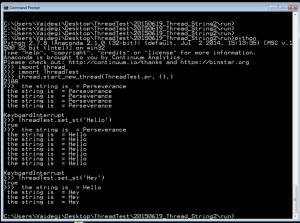### Programming the Deek Robot

The Deek robot is similar to the Arduino pro mini with a ATmega 328. it has no USB interface. i used the Arduino UNO to program the Deek robot, without removing the micro controller ATmega 328 from the UNO board.

1. Connect the Arduino UNO and upload the program

2. Connect jumpers as follows
Arduino      UNO               –>         pro mini
RESET             –>         GRN
RX                   –>         RXD
TX                   –>         TXD
5V                   –>         VCC
GND               –>         GND

GND             –>           BLK

Arduino      UNO               –>         DEEK_ROBOT
RESET             –>         RESET
TX                   –>         TXD
5V                   –>         VCC
GND               –>         GND

GND               –>         GRD

Now using Arduino UNO as an ISP, from the Tools, Programmer .

and selecting the board as Arduino Pro or Pro mini (5V,16Mhz)w ATmega 328, from the Tools, Board.

USING FTDI to program;

FTDI                –>         DEEK_ROBOT
RX                   –>         TXD
TX                   –>         RXD
5V                   –>         VCC
GND               –>         GND

Dt                   –>         DTX

Now  use AVR ISP, from the Tools, Programmer .

and selecting the board as Arduino Pro or Pro mini (5V,16Mhz)w ATmega 328, from the Tools, Board.

### Tabulating the obtained values

The frequency and the sine wave was set by the function generator. The input, output wave forms were observed from the CRO. The conditions taken were  a 50KΩ , 1KΩ and a current source. The input was fed to the 2.1 speaker’s connector jack to produce the sound of the fed sine wave.

 Frequency (Hz) Input (peak to peak value) Conditions Output (Peak to peak value) Observations 600 2 V 50 KΩ 0.2 V Distorted wave 600 2 V 1 KΩ 2 mV Small signal with distorted wave 600 2 V Current Source 100 mV Distorted wave 600 5.5 V Current Source 300 mV Not distorted 600 5.5 V 50 KΩ 100 mV Partially distorted 600 5.5 V 1 KΩ 5 mV Bottom of the wave distorted 450 11 V 1KΩ 8 mV Distorted wave 450 11 V 50 KΩ 200 mV Extremely distorted wave

From the tabulation the maximum output 300mV is achieved when the input is set to a peak to peak value of 5.5V connected from the current source (PNP BJT’s collector junction ).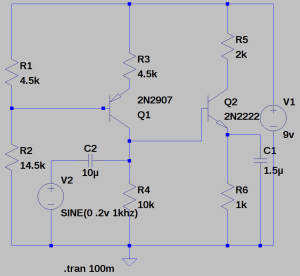To determine the amplification,

the voltage at the base of the NPN transistors is 2.5V. now divided by the resistance of resistor R6 gives us the current.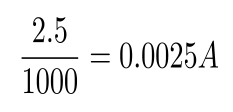now, the obtained current value multiplied by R5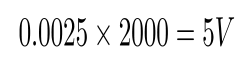gives the amplified output 5V.

### NPN Transistor as an Amplifier :

• We wanted to use the transistor as an amplifier.
• The input voltage at the base was 3v.
• Two resistors, one at the emiiter(R1) and the other at the collector(aR1) were connected.
• 9v supply was given at R1.

Constraints :

• We wanted head room of 2v.
• We wanted to obtain maximum gain.

Design :

• Subtracting 0.7v at the emitter from 3v at the base we get 2.3v.
• Current across R1 is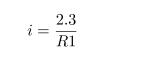• As we needed 2v head room, the voltage drop across aR1 needed to be 4.7v(ie, 4.7v+2.3v=7v) which is 2v lesser then 9v.
• Current across aR1 is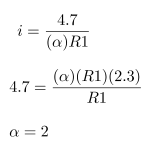• The maximum gain we can get is a gain of 2.
• Hence the resistor value at the collector should be two times more than the one at the emitter.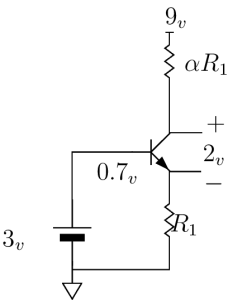### PNP Transistor as a Current Source

• We wanted to use a transistor as a current source.
• Two resistors, one at the emiiter(R1) and the other at the collector(R2) were connected.
• A voltage divider circuit with a supply of 9v was connected at the base that could supply certain voltage.

Constraints :

• We wanted 4.5mA of current to pass through.
• 9v supply was given to the resistor at the emitter.

Design :

• The resistor at the collector(R2) was chosen as 1k based upon the resistance of the mic.
• We knew that the diode would turn on at around 0.7v.
• The voltage drop across R1 should be some resistance that gives 4.5mA of current.
• Depending upon the availability of the resistors, 330 ohms was chosen for R1, keeping the 9v supply in mind and voltage at emiiter, it was calculated that 1.5v drop would give us 4.5mA of current.
• Now from the voltage divider, a certain amount of voltage needed to be supplied so that the drop across R1 is 1.5v (ie, 9v – 7.5v)
• So we wanted 6.8v from the voltage divider (ie, 6.8v + 0.7v = 7.5v)
• Hence, the voltage divider circuit was designed with the following formula.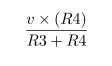• The ratio of the scaling factor was approximately 3 and depending upon the availability of the resistors, R3 was taken as 4.7k and R4 was taken as 14.5k.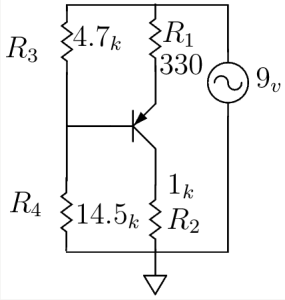### Amplifier – Simulation and Results

First started with constructing constant current source using PNP transistor with 9V supply voltage.

AMPLIFIER:
Added one more transistor on NPN in the circuit with the same supply voltage of 9V and input signal of 0.2V.

Simulation Result: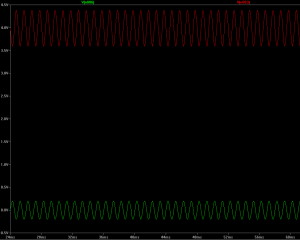Red –> Amplified Output Voltage

Green –> Input Voltage

Schematic diagram:

In order to increase the gain of the Amplifier, added one capacitor C1 parallel with R6 resistor.Red –> Amplified Output Voltage

Green –> Input Voltage

### SpeeDe V_03

SpeeDe V_03:
The aim of the third version of the SpeeDe is to improve the sensitivity to capture the speed of even smaller object. In this regard, I have done some modification in the program ( using the concept of interrupts in Arduino).

ARDUINO PROGRAM:                                                                                                                       // include the library code:
#include <LiquidCrystal.h>
// constants won’t change. They’re used here to
// set pin numbers:
// variables will change:
volatile int State = 0;         // variable for reading the pushbutton status
// Start and stop times
volatile unsigned long start_time_us = 0;
volatile unsigned long stop_time_us = 0;
float delta_time;
float speed_var;

See More

Reference:                                                                                                                             http://www.auraauro.com/uncategorized/speede-v-01-2/
http://arduino.cc/en/Reference/attachInterrupt

### Sampling Theorem

SAMPLING:   A continuous time signal can be represented in its samples and can be recovered back when sampling frequency fs is greater than or equal to the twice the highest frequency (fm) component of message signal. i. e  fs 2fm.

Consider a continuous time signal x(t). The spectrum of x(t) is a band limited to fm Hz. Sampling of input signal x(t) can be obtained by multiplying x(t) with an impulse train δ(t) of period Ts. The output of multiplier is a discrete signal called sampled signal which is represented with y(t) in the following diagrams: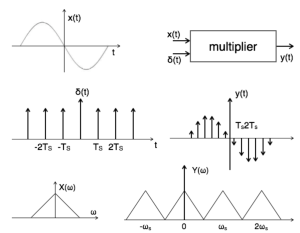ALIASING:

Different signals to become indistinguishable (or aliases of one another) when sampled is called Aliasing. Aliasing occurs when a system is measured at an insufficient sampling rate.Reference:

http://www.tutorialspoint.com/signals_and_systems/signals_sampling_theorem.htm

### Demonstration of Division

Many  teachers struggle with teaching division to children and I have been thinking about this recently. I think there are a number of factors contributing to this. Firstly,  there is a huge range of level of understanding of the concept of division and secondly the concept itself can be thought of as building on children’s understandings of addition, subtraction and multiplication. The spread of different levels of understanding is likely to make any class “mixed ability” for this topic. There are also difficulties with the written algorithm. Altogether, it is a complicated picture. So I Started to create the division program in Scratch to give demonstration.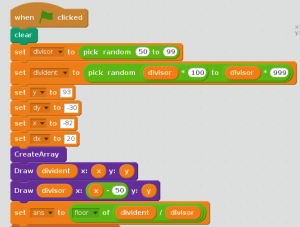See more : https://scratch.mit.edu/projects/56911106/

### At Isai Ambalam

For the first few weeks I decided to teach and learn with the 5th graders at Isai Ambalam middle school, at class we worked out many logic puzzles. The class was at the stage of working out multiplication and division. Speed,distance,time and interrelating each other to form stories of multiplication and division were introduced…

e.g.: if a car travels at a speed of 25 km/hr, what will be the distance covered by the car in 4 hours?

The students multiplied and came up with a solution, by saying that the car would cover a distance of 100kms. The same story was made into division

e.g.: if a car covers 100km in 4hours, what is the speed of the car? The speed of the car is 25km/hr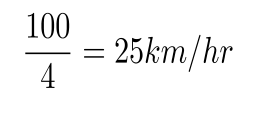the students were able to make out the stories, as we got into creating many different stories I started to realize that not all students did understand what they were making up, some got confused or did not understand the units..

I asked the students how far was their home from school, well there were many loud answers, but each answer terminated with a unit of distance( the lesson learn ‘t was to never ask a general questions to the whole class, or else expect a chorus answer if you don’t want that to happen, be more specific and select a random child to question) . They all knew that time was in hours, but the units of speed and distance always seemed to confuse them until they came to realize that speed is the amount of distance covered over a certain period of time….

the real reason was that the majority of the students did not understand English very well, being bilingual is very very important. But once the conversation starts in Tamil( mother tongue) its easy to forget the conscious of switching back to English…

by the time these few weeks passed the relation with the class grew stronger, I came to realize that there were some four students who seriously did not know what they were doing in mathematics class… every class has a few slow learners, but having students who could not multiply, or even added by using their fingers seemed a little bit odd. I decided to take into consideration these four members of the classroom.

The five of us would sit together and start to solve some multiplication problems, after a while they seemed to get a handle of the multiplication… but the problem showed up in the addition part after multiplying two, two digit numbers… simple additions required finger counting, they could not do mental calculations. If asked what is five plus six they would take five fingers and then start to count six fingers, to see that number 1 was left after adding five plus five and the answer was 11 was not that obvious.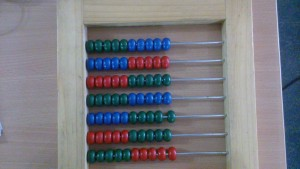To create a change the abacus was introduced and still it was a little hard to see the fives, as the whole ten beads were the same colour. Now the abacus was taken apart and every five beads were altered so as to see the simple pattern of fives. After this, the pattern was seen that when one adds five to seven the remaining number of beads is two and the answer is twelve. To have broken the pattern of finger calculation which they were stuck with for all these years felt awesomely superb. But after a few days with the abacus rigorous training was difficult and the student seemed to be getting a littler tired of beads counting….

that is when introducing scratch programming seemed to ignite a little spark, as a team we came together and built a small script that added two digit numbers. The children were so excited and eager to solve the sums in scratch than before with the abacus. Now doing mental calculations seemed to easier than before.

https://scratch.mit.edu/projects/56560028/